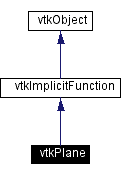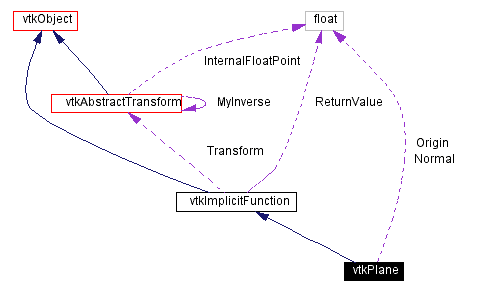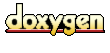Main Page   Class Hierarchy   Alphabetical List   Compound List   File List   Compound Members   File Members   Related Pages

# vtkPlane Class Reference

perform various plane computations. More...

`#include <vtkPlane.h>`

Inheritance diagram for vtkPlane:[legend]
Collaboration diagram for vtkPlane:[legend]
List of all members.

## Public Methods

virtual const char * GetClassName ()
virtual int IsA (const char *type)
void PrintSelf (ostream &os, vtkIndent indent)
float EvaluateFunction (float x)
float EvaluateFunction (float x, float y, float z)
void EvaluateGradient (float x, float g)
virtual void SetNormal (float, float, float)
virtual void SetNormal (float)
virtual float * GetNormal ()
virtual void GetNormal (float data)
virtual void SetOrigin (float, float, float)
virtual void SetOrigin (float)
virtual float * GetOrigin ()
virtual void GetOrigin (float data)

## Static Public Methods

vtkPlane * New ()
int IsTypeOf (const char *type)
vtkPlane * SafeDownCast (vtkObject *o)
void ProjectPoint (float x, float origin, float normal, float xproj)
void ProjectPoint (double x, double origin, double normal, double xproj)
void GeneralizedProjectPoint (float x, float origin, float normal, float xproj)
float Evaluate (float normal, float origin, float x)
float Evaluate (double normal, double origin, double x)
float DistanceToPlane (float x, float n, float p0)
int IntersectWithLine (float p1, float p2, float n, float p0, float &t, float x)

## Protected Methods

vtkPlane ()
~vtkPlane ()
vtkPlane (const vtkPlane &)
void operator= (const vtkPlane &)

float Normal 
float Origin 

## Detailed Description

perform various plane computations.

Date:
2000/12/10 20:08:14
Revision:
1.41

vtkPlane provides methods for various plane computations. These include projecting points onto a plane, evaluating the plane equation, and returning plane normal. vtkPlane is a concrete implementation of the abstract class vtkImplicitFunction.

Examples:
vtkPlane (examples)

Definition at line 61 of file vtkPlane.h.

## Constructor & Destructor Documentation

 vtkPlane::vtkPlane ( ) ` [protected]`

 vtkPlane::~vtkPlane ( ) ` [inline, protected]`
 Definition at line 120 of file vtkPlane.h.

 vtkPlane::vtkPlane ( const vtkPlane & ) ` [inline, protected]`
 Definition at line 121 of file vtkPlane.h.

## Member Function Documentation

 vtkPlane* vtkPlane::New ( ) ` [static]`
 Construct plane passing through origin and normal to z-axis. Reimplemented from vtkObject.

 virtual const char* vtkPlane::GetClassName ( ) ` [virtual]`
 Return the class name as a string. This method is defined in all subclasses of vtkObject with the vtkTypeMacro found in vtkSetGet.h. Reimplemented from vtkImplicitFunction.

 int vtkPlane::IsTypeOf ( const char * type ) ` [static]`
 Return 1 if this class type is the same type of (or a subclass of) the named class. Returns 0 otherwise. This method works in combination with vtkTypeMacro found in vtkSetGet.h. Reimplemented from vtkImplicitFunction.

 virtual int vtkPlane::IsA ( const char * type ) ` [virtual]`
 Return 1 if this class is the same type of (or a subclass of) the named class. Returns 0 otherwise. This method works in combination with vtkTypeMacro found in vtkSetGet.h. Reimplemented from vtkImplicitFunction.

 vtkPlane* vtkPlane::SafeDownCast ( vtkObject * o ) ` [static]`
 Will cast the supplied object to vtkObject* is this is a safe operation (i.e., a safe downcast); otherwise NULL is returned. This method is defined in all subclasses of vtkObject with the vtkTypeMacro found in vtkSetGet.h. Reimplemented from vtkImplicitFunction. Referenced by vtkPlaneCollection::GetNextItem().

 void vtkPlane::PrintSelf ( ostream & os, vtkIndent indent ) ` [virtual]`
 Methods invoked by print to print information about the object including superclasses. Typically not called by the user (use Print() instead) but used in the hierarchical print process to combine the output of several classes. Reimplemented from vtkImplicitFunction.

 float vtkPlane::EvaluateFunction ( float x ) ` [virtual]`
 Evaluate plane equation for point x. Reimplemented from vtkImplicitFunction.

 float vtkPlane::EvaluateFunction ( float x, float y, float z ) ` [inline]`
 Reimplemented from vtkImplicitFunction. Definition at line 72 of file vtkPlane.h.

 void vtkPlane::EvaluateGradient ( float x, float g ) ` [virtual]`
 Evaluate function gradient at point x. Reimplemented from vtkImplicitFunction.

 virtual void vtkPlane::SetNormal ( float , float , float ) ` [virtual]`
 Set/get plane normal. Plane is defined by point and normal.

 virtual void vtkPlane::SetNormal ( float  ) ` [virtual]`

 virtual float* vtkPlane::GetNormal ( ) ` [virtual]`

 virtual void vtkPlane::GetNormal ( float data ) ` [virtual]`

 virtual void vtkPlane::SetOrigin ( float , float , float ) ` [virtual]`
 Set/get point through which plane passes. Plane is defined by point and normal.

 virtual void vtkPlane::SetOrigin ( float  ) ` [virtual]`

 virtual float* vtkPlane::GetOrigin ( ) ` [virtual]`

 virtual void vtkPlane::GetOrigin ( float data ) ` [virtual]`

 void vtkPlane::ProjectPoint ( float x, float origin, float normal, float xproj ) ` [static]`
 Project a point x onto plane defined by origin and normal. The projected point is returned in xproj. NOTE : normal assumed to have magnitude 1.

 void vtkPlane::ProjectPoint ( double x, double origin, double normal, double xproj ) ` [static]`

 void vtkPlane::GeneralizedProjectPoint ( float x, float origin, float normal, float xproj ) ` [static]`
 Project a point x onto plane defined by origin and normal. The projected point is returned in xproj. NOTE : normal does NOT have to have magnitude 1.

 float vtkPlane::Evaluate ( float normal, float origin, float x ) ` [inline, static]`
 Quick evaluation of plane equation n(x-origin)=0. Definition at line 129 of file vtkPlane.h.

 float vtkPlane::Evaluate ( double normal, double origin, double x ) ` [inline, static]`
 Definition at line 134 of file vtkPlane.h.

 float vtkPlane::DistanceToPlane ( float x, float n, float p0 ) ` [inline, static]`
 Return the distance of a point x to a plane defined by n(x-p0) = 0. The normal n must be magnitude=1. Definition at line 140 of file vtkPlane.h.

 int vtkPlane::IntersectWithLine ( float p1, float p2, float n, float p0, float & t, float x ) ` [static]`
 Given a line defined by the two points p1,p2; and a plane defined by the normal n and point p0, compute an intersection. The parametric coordinate along the line is returned in t, and the coordinates of intersection are returned in x. A zero is returned if the plane and line are parallel.

 void vtkPlane::operator= ( const vtkPlane & ) ` [inline, protected]`
 Definition at line 122 of file vtkPlane.h.

## Member Data Documentation

 float vtkPlane::Normal` [protected]`
 Definition at line 124 of file vtkPlane.h.

 float vtkPlane::Origin` [protected]`
 Definition at line 125 of file vtkPlane.h.

The documentation for this class was generated from the following file:

Generated on Wed Nov 21 12:57:20 2001 for VTK by1.2.11.1 written by Dimitri van Heesch, © 1997-2001